### An Overview of RNN

Recurrent Neural Networks (RNN) are another specialized scheme of Neural Network Architectures. RNNs are particularly tuned for time-series or sequential tasks, they are developed to solve learning problems where information about the past (i.e., past instances/ events) are directly linked to making future predictions. Such sequential examples play-up frequently in many real-world tasks such as language modelling where the previous words in the sentence are used to determine what the next word will be. Also in stock market prediction, the last hour/day/week’s stock prices define the future stock movement.

In a sequential problem, there is a looping or feedback framework that connects the output of one sequence to the input of the next sequence. RNNs are ideal for processing 1-Dimensional sequential data, unlike the grid-like 2-Dimensional image data in Convolutional Neural Networks.

This feedback framework enables the network to incorporate information from past sequences or from time-dependent datasets when making a prediction. In this section, we will cover the broad conceptual overview of Recurrent Neural Networks and in particular, the Long Short-Term Memory RNN variant which is the state-of-the-art technique for various sequential problems such as image captioning, stock market prediction, machine translation, and text classification.

### The Recurrent Neuron

The first building block of the RNN is the Recurrent neuron. The neurons of the recurrent network are quite different from those of other neural network architectures. The key difference here is that the Recurrent neuron maintains a memory or a state from past computations. It does this by taking as input the output of the previous instance, $y_{t-1}$ in addition to its current input at a particular instance $x_{t}$.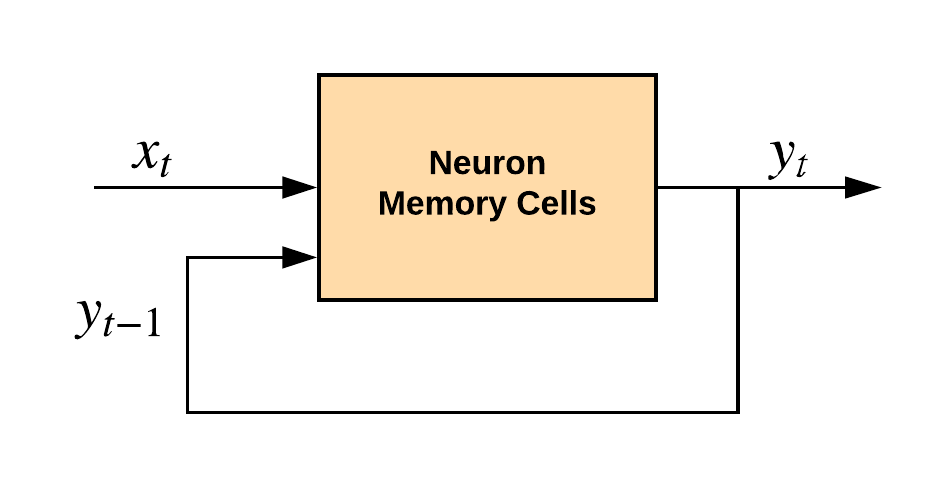Figure 1: A Recurrent Neuron.

From the above image, the recurrent neuron stands in contrast with neurons of the MLP and CNN architectures because instead of transferring a hierarchy of information across the network from one neuron to the other, data is looped back into the same neuron at every new time instance. A time instance can also mean a new sequence.

Hence the recurrent neuron has two input weights, $W_{x_{t}}$ and $W_{y_{t-1}}$ for the input at time $x_{t}$ and for the input at time instance $y_{t-1}$.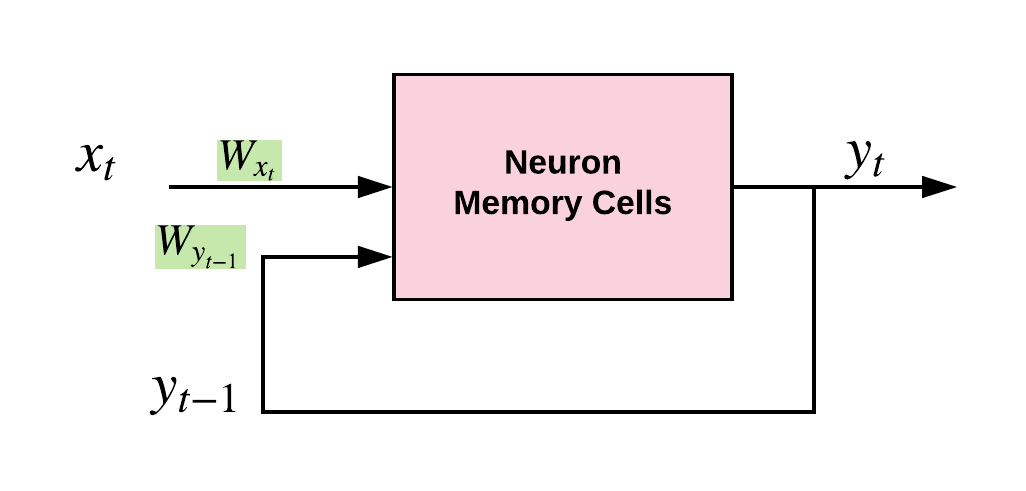Figure 2: Recurrent Neuron with input weights.

Similar to other neurons, the recurrent neuron also injects non-linearity into the network by passing its weighted sums or affine transformations through a non-linear activation function.

### Unfolding the Recurrent Computational Graph

A Recurrent Neural Network is formalized as an unfolded computational graph. An unfolded computational graph shows the flow of information through the recurrent layer at every time instance in the sequence. Suppose we have a sequence of 5 instances, we will unfold the recurrent neuron five times, across the number of instances. The number of sequences constitutes the layers of the recurrent neural network architecture.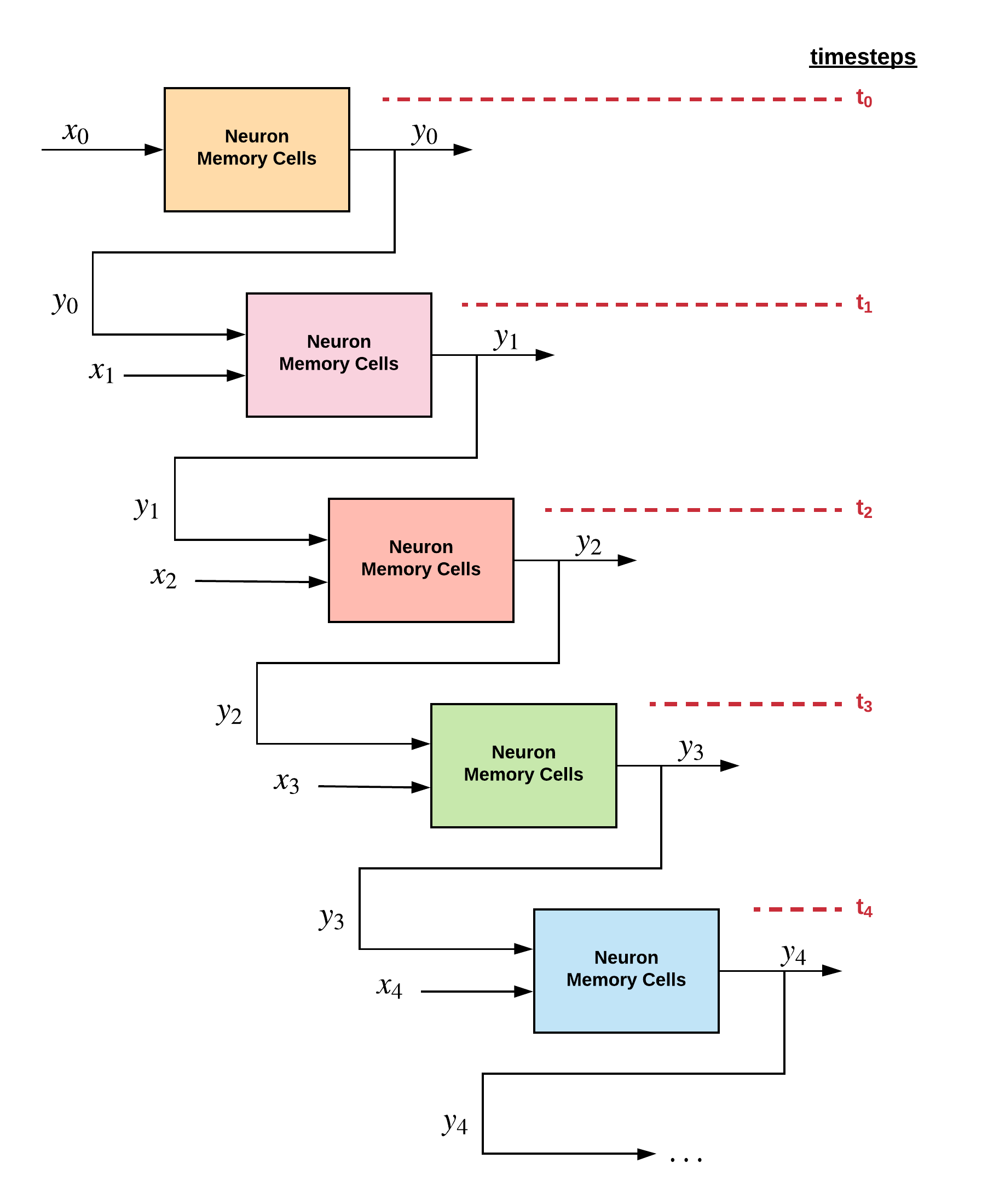Figure 3: Unfolding the Recurrent Neuron into a Recurrent Neural Network.

From the unrolled graph of the recurrent neural network, it is more clearly observed how the input into the recurrent layer includes the output of the previous time step, $t-1$ in addition to the current input at time step $t$. This architecture of the recurrent neuron is central to how the recurrent neural network learns from past events or past sequences.

Up until now, we have noticed that the recurrent neuron captures information from the past, by storing memory or state in its memory cell. The recurrent neuron can have a much more complicated memory cell (such as the GRU or LSTM cell) than the basic RNN cell as illustrated in the images so far, where the output at time instance $t-1$ holds the memory.

### Basic Recurrent Neural Network

Earlier on, we mentioned that when a recurrent network is unfolded, we can see how information flows from one recurrent layer to the other. And we noted that the sequence length of the dataset determines the number of recurrent layers. Let’s briefly illustrate this point. Suppose we have a time series dataset of 10 layers, for each row sequence in the dataset, we will have 10 layers in the recurrent network system.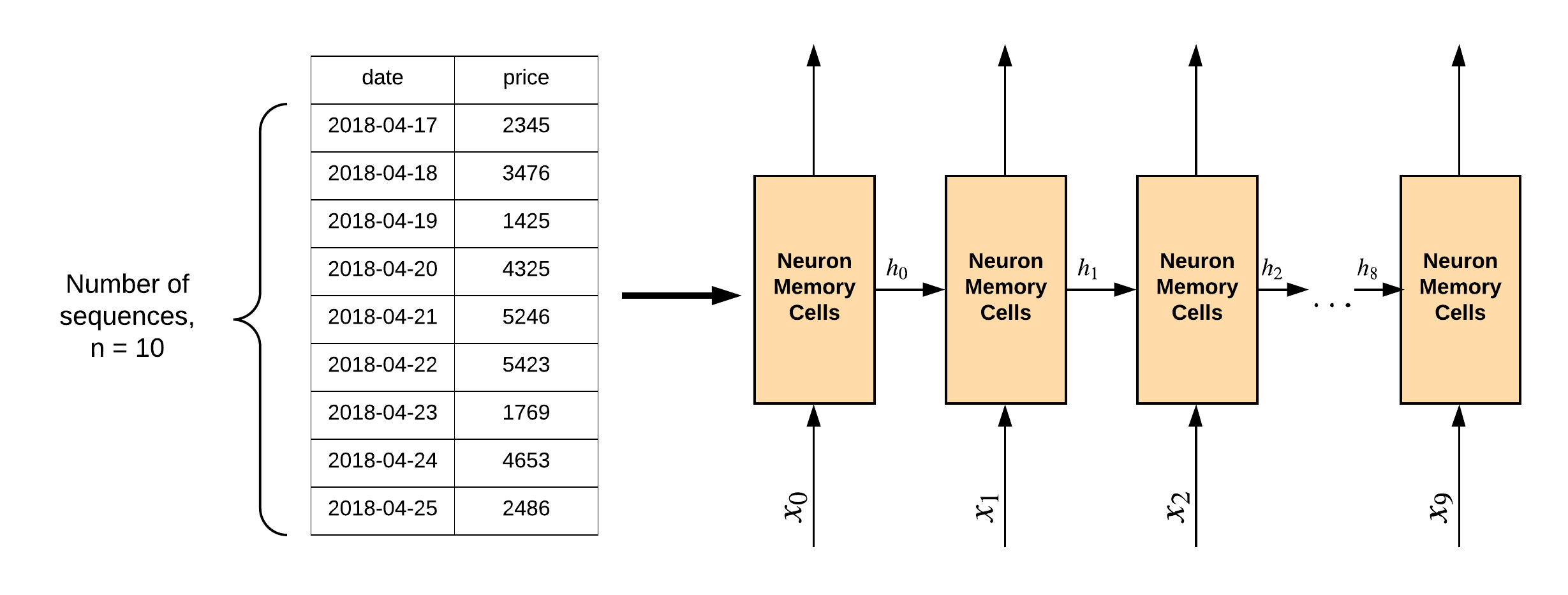Figure 4: Dataset to Layers.

At this point, we must firmly draw attention to the fact that the recurrent layer does not comprise of just one neuron cell, but it is rather a set of neurons or neuron cells. The choice of the number of neurons in a recurrent layer is a design decision when composing the network architecture.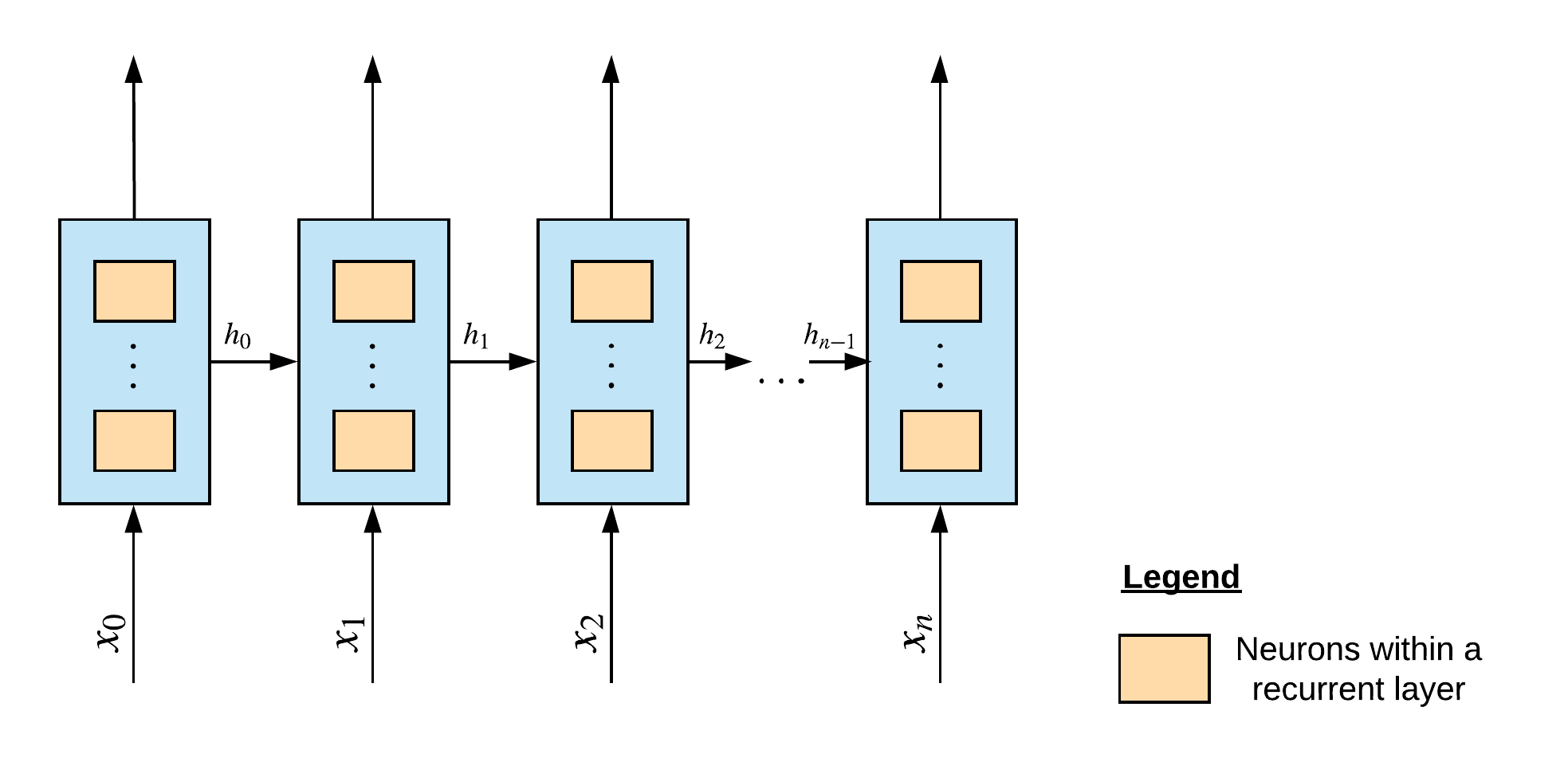Figure 5: Neurons in a recurrent layer.

Each neuron in a recurrent layer receives as input the output of the previous layer, and it’s current input. Hence, the neurons each have two weight vectors. Again, just like other neurons, they perform an affine transformation of the inputs and pass it through a non-linear activation function (usually the hyperbolic tangent, tanh). Still, within the recurrent layer, the output of the neurons are moved to a dense or fully connected layer with a softmax activation function for outputting the class probabilities. This operation is illustrated below: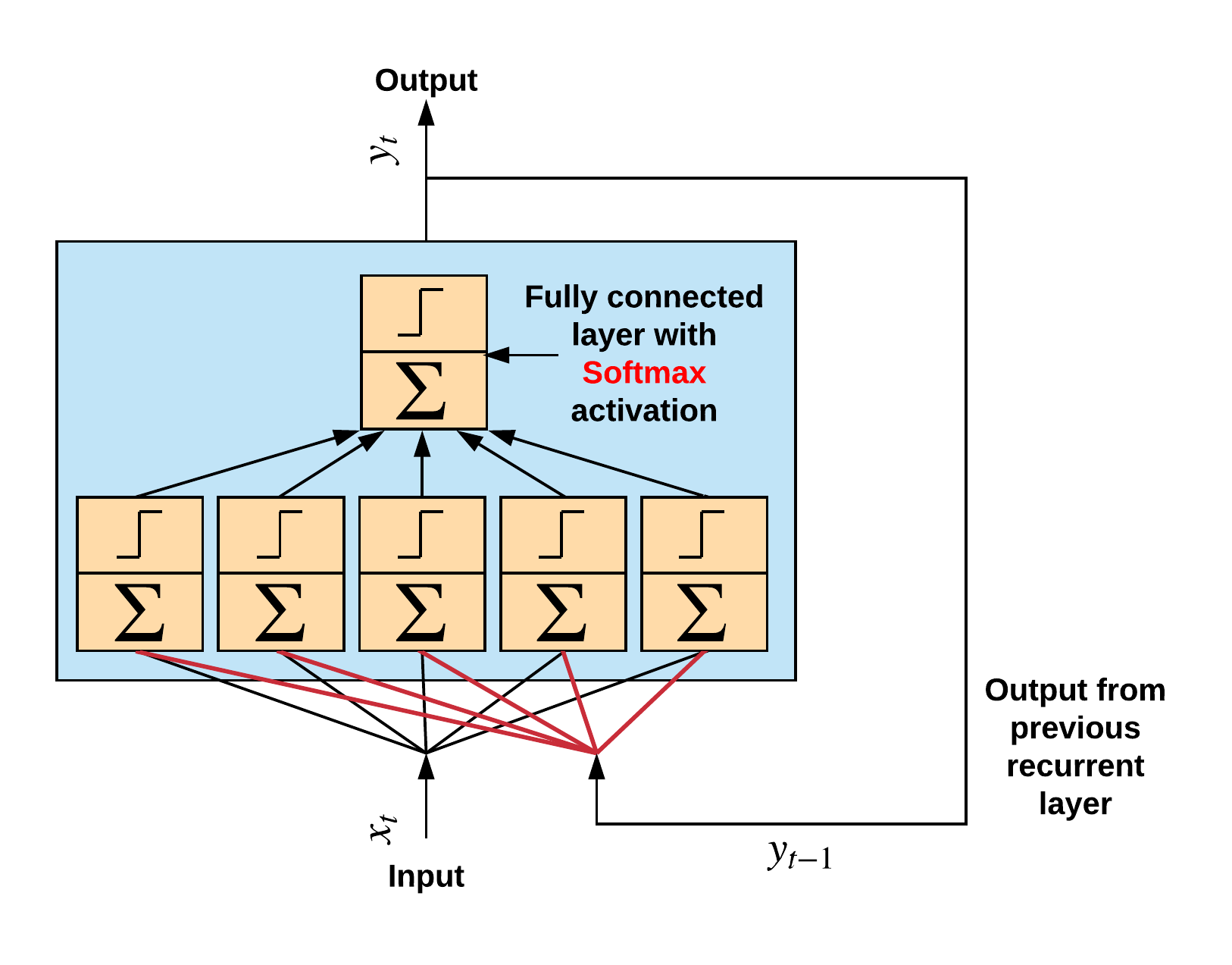Figure 6: Computations within a Recurrent Layer.

### Recurrent Connection Schemes

There are two main schemes for forming recurrent connections from one recurrent layer to another. The first is to have recurrent connections between hidden units, and the other is recurrent connections between the hidden unit and the output of the previous layer. The different schemes are visually illustrated below: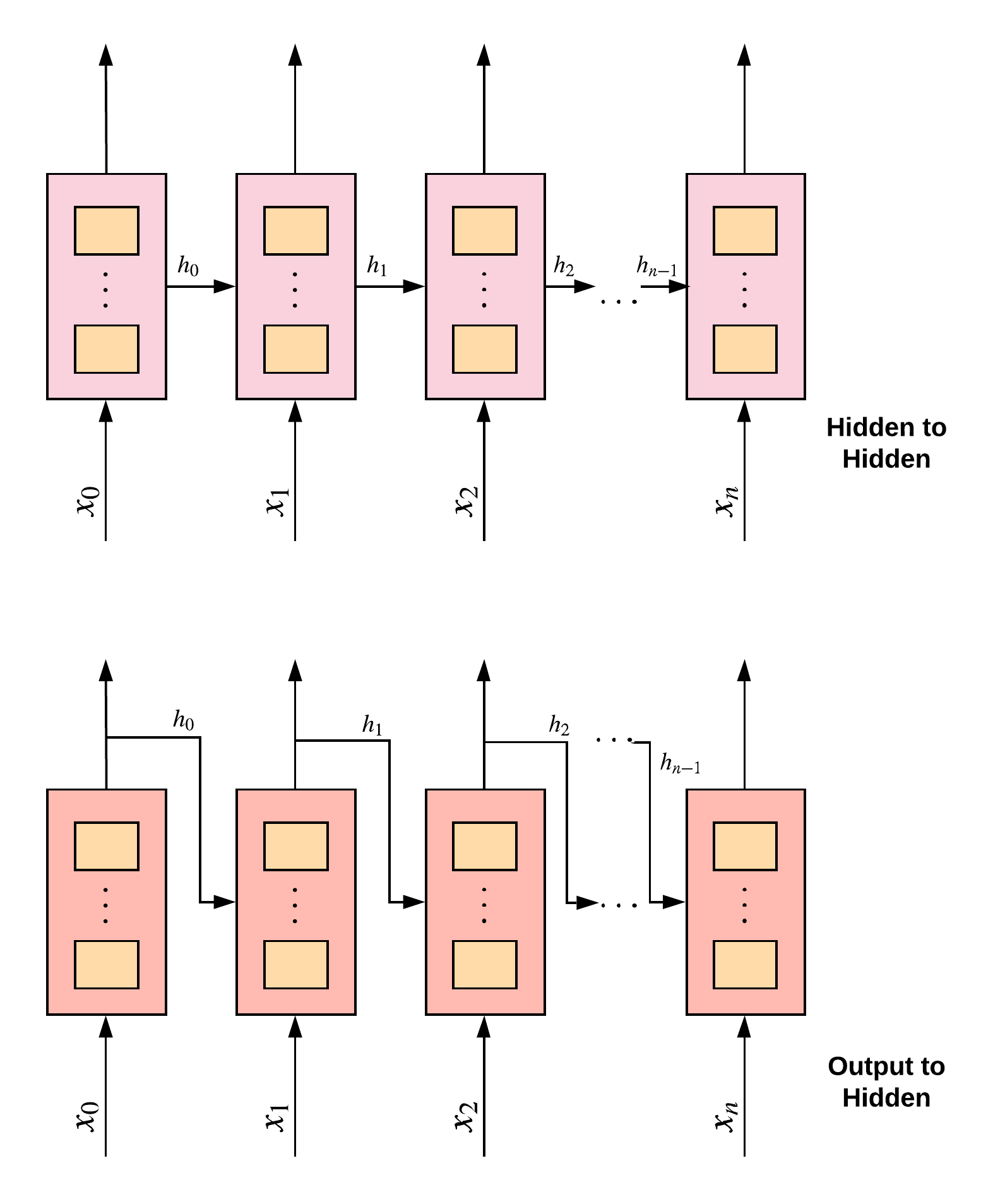Figure 7: Recurrent Connection Schemes.

The hidden-to-hidden recurrent configuration is found to be superior to the output-to-hidden form because it better captures the high-dimensional feature information about the past. In any case, the output-to-hidden recurrent form is less computationally expensive to train and can more easily be parallelized.

### Training the Recurrent Network: Backpropagation Through Time

The Recurrent Neural Network is trained in much the same way as other traditional neural networks - by using the Backpropagation algorithm. However, the Backpropagation algorithm is modified into what is called Backpropagation Through Time (BPTT).

Due to the architectural loop or recurrent structure of the recurrent network, vanilla Backpropagation as-is cannot work. Training a network using backpropagation involves calculating the error-gradient, moving backward from the output layer through the hidden layers of the network and adjusting the network weights. But this operation cannot work in the Recurrent neuron because we have just one neural cell with recurrent connections to itself.

So in order to train the Recurrent network using Backpropagation, we unroll the Recurrent neuron across the time instances and apply backpropagation to the unrolled neurons at each time layer the same way it is done for a traditional feedforward neural network. This operation is further illustrated in the image below.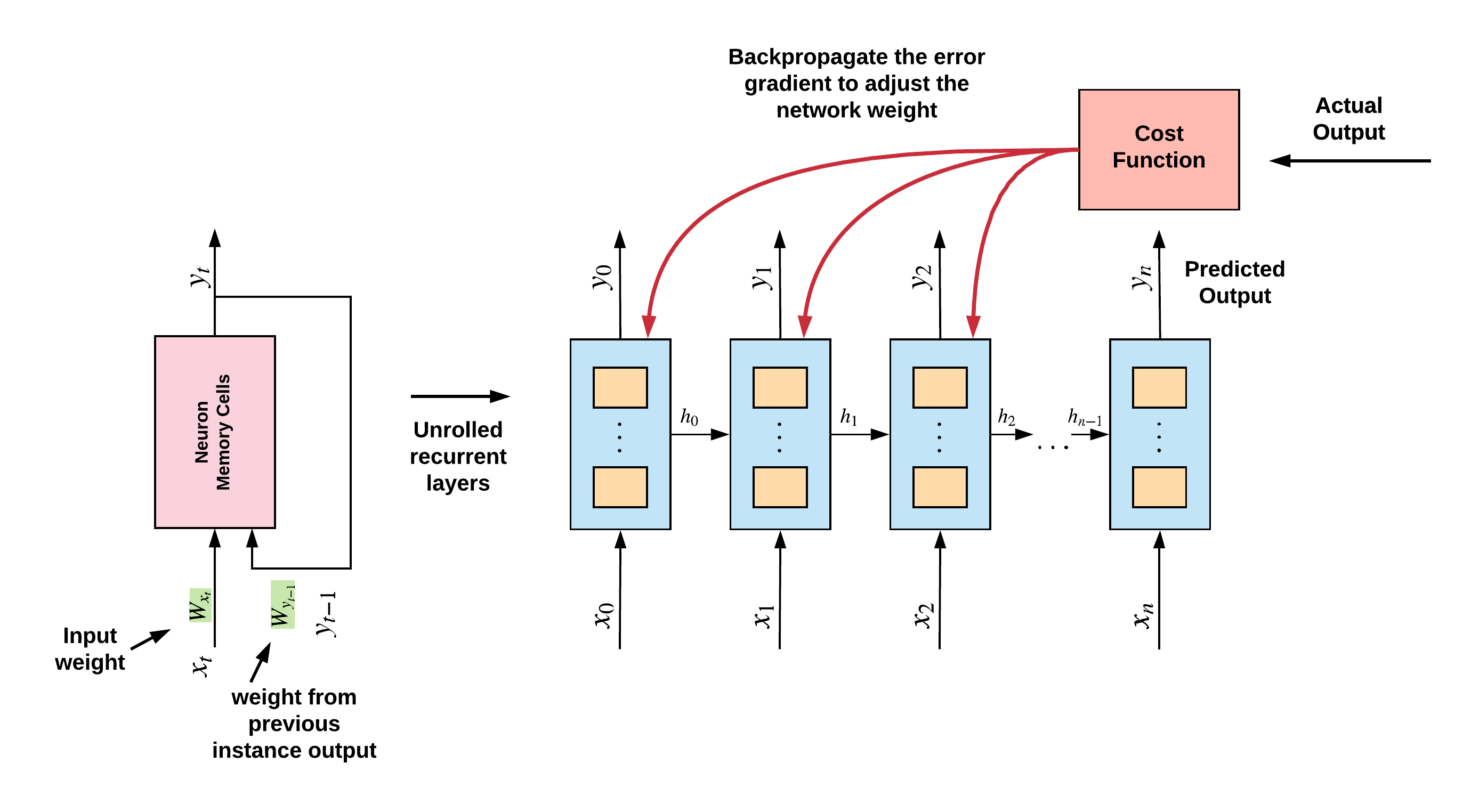Figure 8: Backpropagation Through Time.

A significant challenge of training the Recurrent neural network is the vanishing and exploding gradient problem. When training a deep recurrent network for many layers of time instances, calculating the gradients of the weights of the neurons can become very volatile. When this happens, the value of the gradient can become extremely large tending to infinity, or they become tiny, all the way to zero. When this happens, the neurons become dead, and cannot train or learn any new information further. This effect is called the exploding and vanishing gradient problem.

The exploding and vanishing gradient problem is most prevalent in Recurrent Neural Networks because of the long-term dependencies or time instance of the unrolled recurrent neuron. A proposed alternative technique for mitigating this problem in Recurrent networks (in addition to other discussed methods such as Gradient clipping, Batch Normalization and using a non-saturating activation function such as ReLu), is to discard early time instances or time instances in the distant past. This technique is called Truncated Backpropagation Through Time (BPTT).

However, BPTT suffers a major drawback, and this is that some problems rely heavily on long-term dependencies to be able to make a prediction. A typical example is in language modelling where the long-term sequence of words in the past is vital in predicting the next word in the sequence.

The short-coming of BPTT and the need to deal with the problem of exploding and vanishing gradients led to the development of a memory cell called the Long-Short Term Memory or LSTM for short, which can store the long-term information of the problem in the memory cell of the recurrent network.

### The Long Short Term Memory (LSTM) Network

Long Short-Term Memory (LSTM) belongs to a class of RNN called Gated recurrent unit. They are called gated because unlike the basic recurrent units, they contain extra components called gates that control the flow of information within the recurrent cell. This includes choosing what information to store in the cell, and what information to discard or forget.

LSTM is very efficient for capturing the long-term dependencies across a large number of time instances. It does this by having a slightly more sophisticated cell than the basic recurrent units. The components of the LSTM are the:

• Memory cell,
• Input gate,
• Forget gate,
• Output gate

These extra components enable the RNN to remember and store important events from the distant past. The LSTM takes as input, the previous cell state, $c_{t-1}$ the previous hidden state, $h_{t-1}$ and the current input, $x_{t}$. To keep in line with the simplicity of this material, we provide a high-level illustration of the LSTM cell showing how the extra components of the cell come together.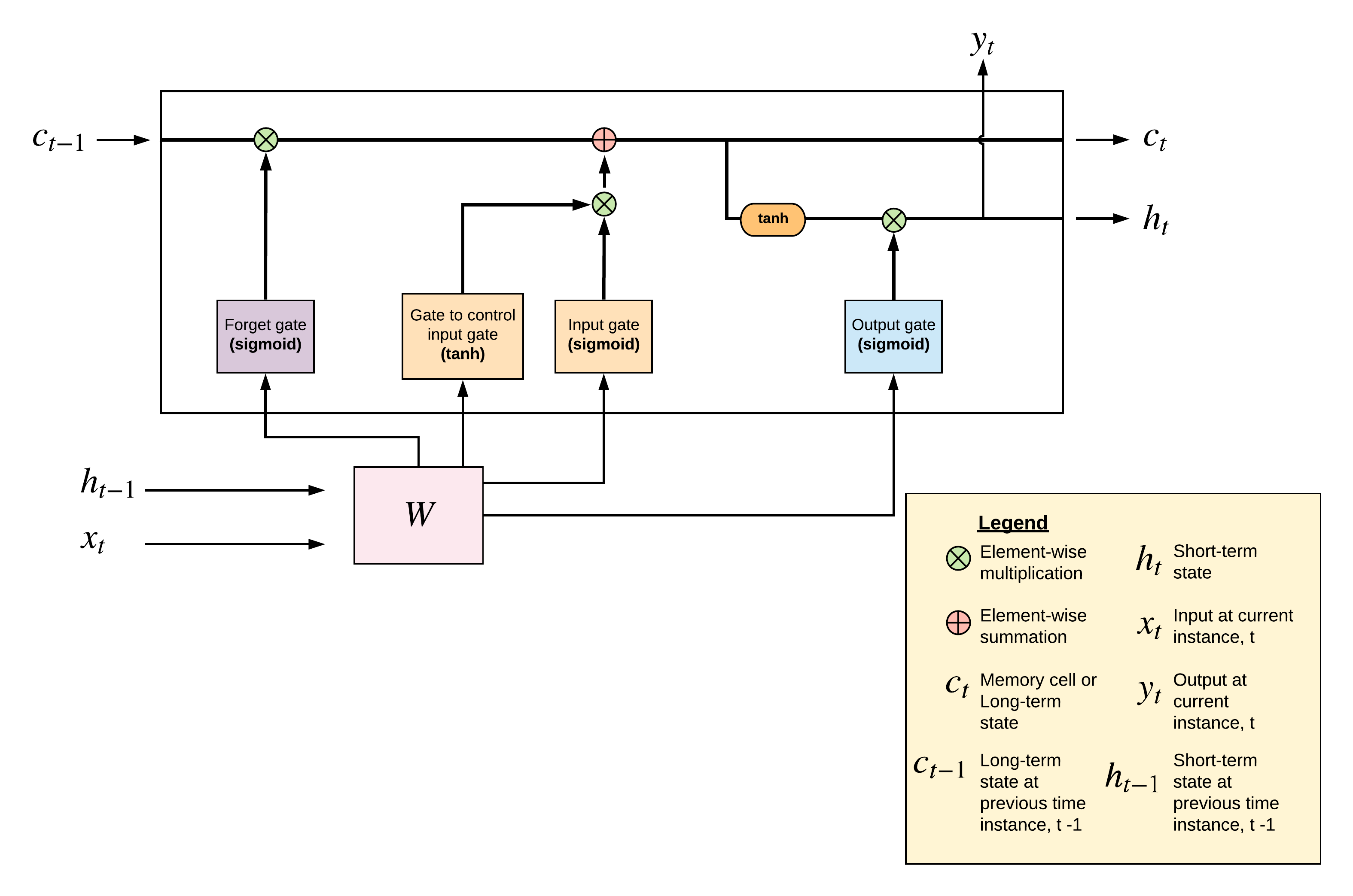Figure 9: LSTM Cell.

The illustration in Figure 9 is the LSTM memory cell. The components of the LSTM cell serve distinct functions in preserving long-term dependencies in sequence data. Let’s go through them.

• The Input gate: This gate is responsible for controlling what information gets stored in the long-term state or the memory cell, $c$. Working in tandem with the input gate is another gate that regulates the information flowing into the input gate. This gate analyzes the current input to the LSTM cell, $x_{t}$, and the previous short-term state, $h_{t-1}$.
• The Forget gate: The role of this gate is to regulate how much of the information in the long-term state is persisted across time instances.
• The Output gate: This gate controls how much information to output from the cell at a particular time instance. This gate controls the value of $h_{t}$ (the short-term state) and $y_{t}$ (the output at time $t$).

It is important to note that the components of the LSTM cells are all Fully-Connected Neural Networks. There exist other variants of Recurrent Networks with memory cells, two of such are the peephole connections and the Gated Recurrent Units.